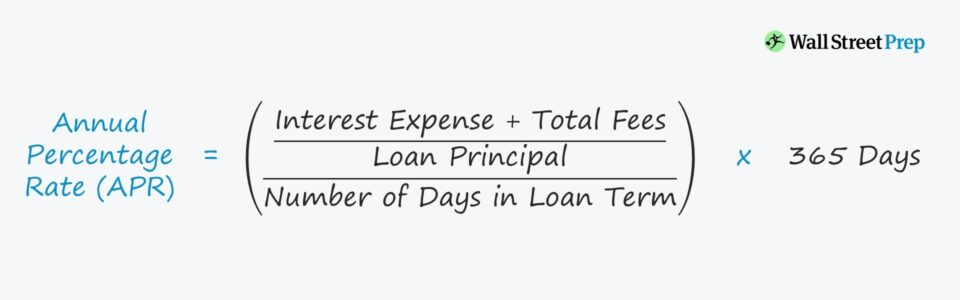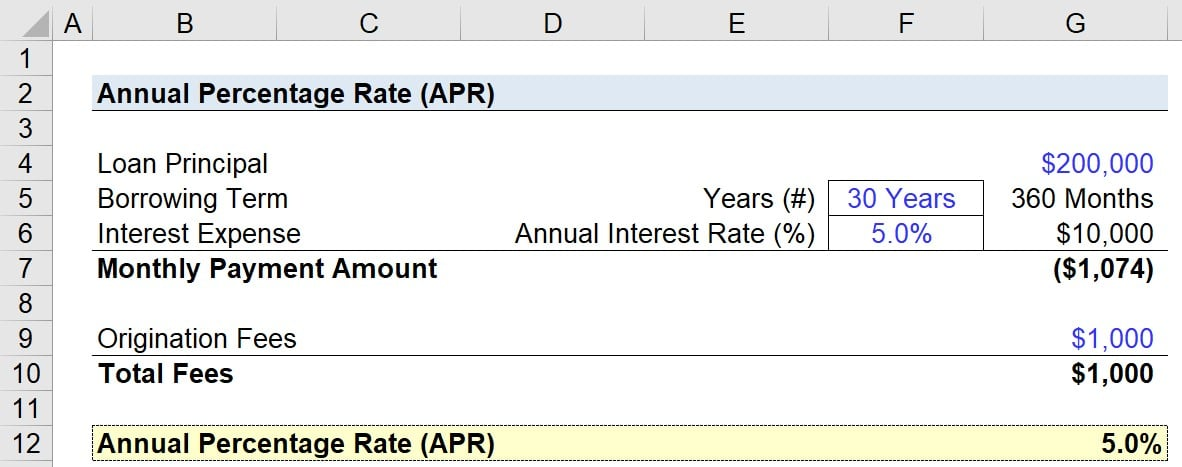Welcome to Wall Street Prep! Use code at checkout for 15% off.# Annual Percentage Rate (APR)

Guide to Understanding Annual Percentage Rate (APR)## How to Calculate APR?

The APR, or “annual percentage rate”, is defined as the interest rate paid each year on an outstanding loan amount.

Conceptually, APR represents the estimated cost of the yearly fees associated with a specific type of borrowing.

APR is a standard calculation used regularly by lenders, as the financial metric is designed to help borrowers understand the implied returns and compare different loan options.

The stated interest rate on a loan is not usually enough on its own to make the right borrowing decision – for instance:

1. A loan with a low stated interest rate might come with substantial fees which cannot be neglected.
2. A loan with a higher stated interest rate, yet lower fee structure could be the preferable option.

## APR Formula

The annual percentage rate (APR) is calculated using the following formula.

Annual Percentage Rate (APR) = (Periodic Interest Rate x 365 Days) x 100

Where:

• Periodic Interest Rate = [(Interest Expense + Total Fees) / Loan Principal] / Number of Days in Loan Term

To express the APR as a percentage, the amount must be multiplied by 100.

## What is the Conceptual Meaning of APR?

The annual percentage rate (APR) on a loan – under a mortgage financing scenario, for example – marks the total yearly cost associated with borrowing money from a financial institution.

Since more fees beyond just interest expenses are considered in the APR of a loan, the metric provides a more accurate estimation of how much in total a borrower must pay to take out a loan.

The APR on loans facilitates comparisons across different loan offerings (i.e. for the borrower to pick the cheapest option), yet in actuality, the comparison is not “apples-to-apples” due to several factors:

• Oftentimes, loan tranches can be “taken out” (i.e. repaid in full earlier than scheduled) or refinanced before the date of maturity.
• Standardizing the fees charged by the lender is practically impossible (i.e. different types per financing arrangement).
• Contingencies can be influential factors such as prepayment penalties, conditional fees, and incentive programs.

## APR Lending: Financing and Fee Examples

The annual percentage rate (APR) is a relevant concept for numerous financing scenarios, most notably:

• Mortgage
• Consumer and Commercial Loans
• Line of Credit (LOC)
• Credit Card
• Auto Loans

Depending on the specific circumstances of the financing, the additional fees incurred on the side could include:

• Underwriting Fees
• Origination Fees
• Processing Fees
• Appraisal Fees

## What is APR on a Credit Card?

Under the context of credit cards, the annual percentage rate (APR) determines the amount of interest due based on the carrying balance from month to month.

If each monthly bill is paid in full and on time, no interest is incurred, since the obligation is met.

Unique to credit cards, interest is calculated daily, meaning that a credit card company charges borrowers by multiplying the ending balance by the APR and then dividing by 365.

The amount of interest charged is subsequently added to the outstanding balance the following day.

In contrast to credit cards, the APR on a loan reflects more than just the interest payments that must be met.

## What is the Difference Between APR vs. APY?

One common mistake is to confuse the annual percentage rate (APR) with the annual percentage yield (APY).

To distinguish between the two finance terms, the annual percentage rate (APR) is the interest that a borrower must pay on a loan, whereas the annual percentage yield (APY) is the interest a lender would expect to earn on an investment.

• Annual Percentage Rate (APR) ➝ Interest “Owed”
• Annual Percentage Yield (APY) ➝ Interest “Earned”

APR is also an annualized simple interest rate, while the APY calculation considers the effects of compounding.

As a general rule, the higher the interest rate and the fewer compounding periods there are, the greater the discrepancy between the annual percentage rate (APR) and the annual percentage yield (APY).

## What is the Difference Between Fixed vs. Variable APR?

The final difference we’ll explain is between a fixed APR and a variable APR:

A fixed APR is thus more predictable than a variable APR, which is a function of the market conditions and the specific benchmark by which its value is influenced.

## APR Calculator

We’ll now move to a modeling exercise, which you can access by filling out the form below.Submitting...

## 1. Mortgage Loan and Interest Rate Assumptions

Suppose you’ve taken out a mortgage loan with the following lending terms:

• Mortgage Amount: \$200,000
• Lending Term: 30 Years, or 360 Months
• Interest Rate (Annual): 5%

Remember, APR does not just factor in the interest expense, but related fees, too.

• Origination Fee: \$1,000

## 2. APR Calculation Example (PMT and RATE Excel Function)

Using the “PMT” function in Excel, we can calculate the monthly payment amount.

=PMT (Interest Expense / 12, Borrowing Term in Months, Loan Principal)

If we plug in our numbers, we get the following:

• Monthly Payment = PMT (\$10,000 / 12, 360, \$200,000)
• Monthly Payment = \$1,074

The “RATE” Excel function can then be utilized to arrive at our mortgage’s annual percentage rate (APR).

=RATE (Borrowing Term in Months, Monthly Payment, (Loan Principal – Origination Fee)) * 12

## 3. Annual Percentage Rate Calculation Example (APR)

Since we already have all the required inputs, the only remaining step is to plug them into the Excel formula from earlier.

• APR = RATE (360, \$1,074, (\$200,000 – \$1,000)) * 12

The annual percentage rate (APR) on the mortgage loan comes out to be approximately 5.0%.

• Annual Percentage Rate (APR) = 5.044%Step-by-Step Online Course

### Everything You Need To Master Financial Modeling

Enroll in The Premium Package: Learn Financial Statement Modeling, DCF, M&A, LBO and Comps. The same training program used at top investment banks.Inline FeedbacksGet the WSP Fixed Income Markets Certification (FIMC©)

Wall Street Prep’s globally recognized certification program prepares trainees with the skills they need to succeed as a Fixed Income Trader on either the Buy Side or Sell Side.

X

The Wall Street Prep Quicklesson Series

7 Free Financial Modeling Lessons

Get instant access to video lessons taught by experienced investment bankers. Learn financial statement modeling, DCF, M&A, LBO, Comps and Excel shortcuts.